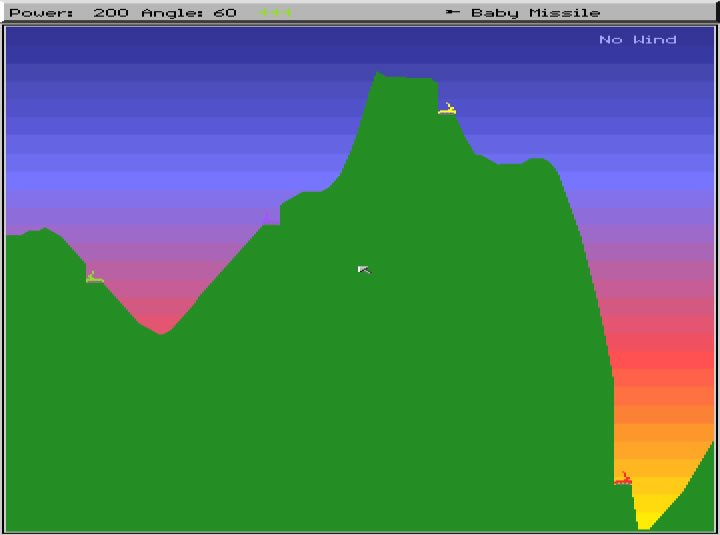#### Midpoint displacement algorithm in c++(make hight map for tank like scorched earth)

midpoint displacement algorithm in c++(make hight map for tank like scorched earth)

now the problem that i cant get it to work properly what can i do?

here what i get when it executed

Result:Expected:note!!!!!!!!!! that the screen y axis is in SDL2 from up to down 0 is up 1000 +- is down

before the function i have the initializing variables:

``````std::vector<int> points(g_SCREEN_WIDTH);
// Gives us a power of 2 based on our width

double Displacement = 100;       //diplacment 1 to 1000
double roughness = 2500;   //between 1 to 500000 more smoother
double itrations = g_SCREEN_WIDTH;
float range = 2;
bool parlien = 0, onlyOne =0;

srand(time(NULL));

int choose;

choose = rand() % 3 + 1;
switch (choose) {
case 1: {Displacement = rand() % g_SCREEN_HEIGHT; break; }
case 2: {Displacement = g_SCREEN_HEIGHT; break; }
case 3: {Displacement = rand(); break; }
}

choose = rand() % 5 + 1;

switch (choose) {
case 1: {roughness = rand() % 250 + 50; break; }
case 2: {roughness = rand() % 500 + 250; break; }
case 3: {roughness = rand() % 2500 + 500; break; }
case 4: {roughness = rand() % 5000 + 2500; break; }
case 5: {roughness = rand() % 50000 + 5000; break; }
break;
}

choose = rand() % 4 + 1;
switch (choose) {
case 1: {itrations = rand() % 64 + 1; break; }
case 2: {itrations = rand() % 150 + 64; break; }
case 3: {itrations = rand() % 265 + 150; break; }
case 4: {itrations = rand() % 1024 + 265; break; }
break;
}

//itrations = 12;
int mountents;
mountents = rand() % 3;
//itrations = 16;

// mountents = 1;

//the first intlising for the points
{
int y = rand() %(int)Displacement;
points.at(0) = y;
y = rand() %(int)Displacement;
points.at(g_SCREEN_WIDTH - 1) = y;
}

//parlien = 0;

Displacement = (g_SCREEN_HEIGHT/8);  //more its little it  smother
roughness = 0.5;        //more its little it going up
itrations = 256;

std::cout << "Displacement:" << Displacement << "n";
std::cout << "roughness:" << roughness << "n";
std::cout << "itrations:" << itrations << "n";
std::cout << " g_SCREEN_WIDTH:" << g_SCREEN_WIDTH << "n";
``````

here is the function

``````int func(double left, double right,std::vector<int> *points, double roughness, double segmnets, double Displacement, double itrations,int mountents,float range)
{
double mid=(left+right)/2;  //it gets the midpoint of the segment we get here
if (Displacement <= 1) Displacement = 1;
//std::cout << "Displacement:" << Displacement << "n";

int y;

if (range < 1) range = 1;
if (mid <= 1) return 1;
if (segmnets > itrations - 1) return 1;
if (segmnets >= g_SCREEN_WIDTH) return 1;

int Choose;

y = -1;
int cnt = 0;
while (y<25 || y>g_SCREEN_HEIGHT)
{
y = ((points->at(left) + points->at(right)) / 2);
float r = static_cast <float> (rand()) / static_cast <float> (RAND_MAX);
y = y + Displacement * r;
y = abs(y);
y= ::g_SCREEN_HEIGHT - y;
cnt++;
if (cnt > 2) { std::cout << "cnt++:" << "n"; y = rand() % (int)Displacement; break; }
}

if (y < 25) y = 25;
if (y > g_SCREEN_HEIGHT) y = g_SCREEN_HEIGHT - 1;

left= mid / 2;
right = mid + (mid / 2);

if (mid > 0 && mid < g_SCREEN_WIDTH)
{
points->at(mid) = y;

}

Displacement /= 2;

func(0,mid, points, roughness, segmnets * 2, Displacement, itrations, mountents,range);

//for the rigths
func(mid, g_SCREEN_WIDTH-1, points, roughness, segmnets * 2, Displacement, itrations, mountents,range);

}
``````

Source: Windows Questions